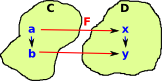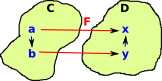# Maths - Functor - Covarient and Contravarient

## Covarient and Contravarient Functors

There are two ways that a given morphism can preserve structure:

 This is the covarient case where the start of the arrow in C maps to the start of the arrow in D and the end of the arrow in C maps to the end of the arrow in D.This is the contravarient case where the start of the arrow in C maps to the end of the arrow in D and the end of the arrow in C maps to the start of the arrow in D.Unless otherwise stated we usually assume that a morphism/functor is covarient so we can draw this as on the left.For the contravarient case we could invent some sort of special symbol for a contravarient morphism/functor. However is usually easier to reverse the internal arrows in either C or D. On the left we have reversed C by making it Cop. So now we can use an ordinary arrow between C and D.

The way that covarient and contravarient functors arise, in this case, is as follows.

Let 'A', 'X' and 'Y' be elements of a class 'C'. let 'f' be a functor from 'X' to 'Y'. Assume that both 'A' and 'f' are fixed:

covarient contravarient• If 'f' and 'h' are known then we can derive 'g' (by composition)
• If 'f' and 'g' are known then we cannot derive 'h' (see concepts of section and retraction).

So given some fixed 'f' the 'g' depends on 'h' which I have drawn below as an arrow between arrows.

• If 'f' and 'g' are known then we can derive 'h' (by composition)
• If 'f' and 'h' are known then we cannot derive 'g' (see concepts of section and retraction).

So given some fixed 'f' the 'h' depends on 'g' which I have drawn below as an arrow between arrows.This arrow goes in the same direction to f, hence it is covarient. This arrow goes in the opposite direction to f, hence it is contravarient.

We now need to combine these ideas with the concept of hom sets as follows.

Where I can, I have put links to Amazon for books that are relevant to the subject, click on the appropriate country flag to get more details of the book or to buy it from them.The Princeton Companion to Mathematics - This is a big book that attempts to give a wide overview of the whole of mathematics, inevitably there are many things missing, but it gives a good insight into the history, concepts, branches, theorems and wider perspective of mathematics. It is well written and, if you are interested in maths, this is the type of book where you can open a page at random and find something interesting to read. To some extent it can be used as a reference book, although it doesn't have tables of formula for trig functions and so on, but where it is most useful is when you want to read about various topics to find out which topics are interesting and relevant to you.